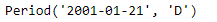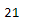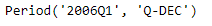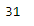# Python | Pandas Period.day

• Last Updated : 06 Jan, 2019

Python is a great language for doing data analysis, primarily because of the fantastic ecosystem of data-centric python packages. Pandas is one of those packages and makes importing and analyzing data much easier.

Pandas` Period.day `attribute returns the day of the month for the given Period object. It returns an integer value.

Syntax : Period.day

Parameters : None

Return : day

Example #1: Use `Period.day` attribute to find the day in the given Period object.

 `# importing pandas as pd``import` `pandas as pd`` ` `# Create the Period object``prd ``=` `pd.Period(freq ``=``'D'``, year ``=` `2001``, month ``=` `1``, day ``=` `21``)`` ` `# Print the Period object``print``(prd)`

Output :Now we will use the `Period.day` attribute to find the day of the month in the given object.

 `# return the day value ``prd.day`

Output :As we can see in the output, the `Period.day` attribute has returned 21 which is the value of day in the given period object.

Example #2: Use `Period.day` attribute to find the day in the given Period object.

 `# importing pandas as pd``import` `pandas as pd`` ` `# Create the Period object``prd ``=` `pd.Period(freq ``=``'Q'``, year ``=` `2006``, quarter ``=` `1``)`` ` `# Print the object``print``(prd)`

Output :Now we will use the `Period.day` attribute to find the day of the month in the given object.

 `# return the day value ``prd.day`

Output :As we can see in the output, the `Period.day` attribute has returned 31 which is the value of day in the given period object.

My Personal Notes arrow_drop_up